# The Deltoidal Icositetrahedron

The deltoidal icositetrahedron is a 3D Catalan solid bounded by 24 kites, 48 edges (12 short, 12 long), and 26 vertices. It is the dual of the rhombicuboctahedron.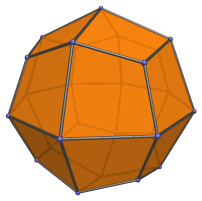The kite faces are transitive, with two short edges and two long edges with a (4+√2) : 7 length ratio.

The 26 vertices are of three kinds: 6 apical vertices corresponding to an inscribed octahedron, 8 vertices corresponding to an inscribed cube, and 12 vertices corresponding to an inscribed cuboctahedron.

## Projections

The following are images of the deltoidal icositetrahedron from various viewpoints:

Projection Description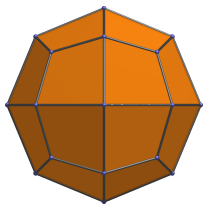Front view, centered on an axial vertex.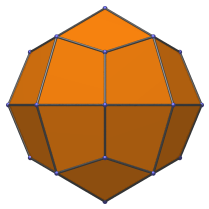Side view, centered on a cuboctahedron vertex.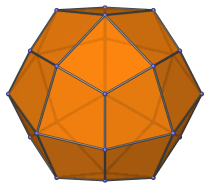Octant view, centered on a cube vertex.

## Animation

Here's an animation of a deltoidal icositetrahedron rotating around the vertical axis: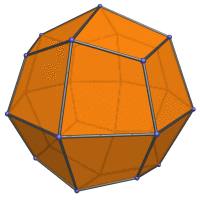## Coordinates

The Cartesian coordinates for the deltoidal icositetrahedron are all permutations of coordinate and changes of sign of:

• (0, 0, (2√2−1))
• (0, (4−√2)/2, (4−√2)/2)
• (1, 1, 1)

These coordinates can be obtained by inverting a rhombicuboctahedron of edge length (3−√2)/7.

Last updated 03 Feb 2023.